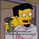1231 views
KDJ indicator
```study("KDJ Indicator")
// KDJ Indicator, Rev 03
//
// Rewrite for Tradingview "Pine Script" by Kocurekc, April 2014
// Code is provided as public domain, no warranty

lenL = input(title="Lookback Period, Long", type=integer, defval=14, minval=2)
lenS = input(title="Lookback Period, Short", type=integer, defval=5, minval=2)
bE = input(title="Use EMA?", type=bool, defval=true)
bJ = input(title="Smooth the %J?", type=bool, defval=false)
lenJ = input(title="%J Smoothing", type=integer, defval=5, minval=1)

s = stoch(close, high, low, lenL)
pK = bE ? ema(s,5) : sma(s,5)
pD = bE ? ema(pK, lenS) : sma(pK, lenS)
pJ = (3 * pD) - (2 * pK)

plot(pK, color=blue)
plot(pD, color=red)
plot(bJ ? sma(pJ,lenJ) : pJ, color=green)```